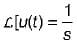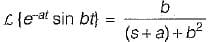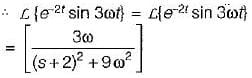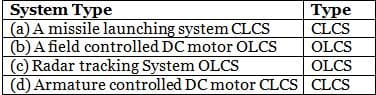# Test: Introduction to Control Systems- 2

## 10 Questions MCQ Test Topicwise Question Bank for Electrical Engineering | Test: Introduction to Control Systems- 2

Description
Attempt Test: Introduction to Control Systems- 2 | 10 questions in 30 minutes | Mock test for Electrical Engineering (EE) preparation | Free important questions MCQ to study Topicwise Question Bank for Electrical Engineering for Electrical Engineering (EE) Exam | Download free PDF with solutions
QUESTION: 1

Solution:
QUESTION: 2

Solution:
QUESTION: 3

### The difference between the output response and the reference signal is known as the _____ signal.

Solution:
QUESTION: 4

The Laplace transform of unity function is

Solution:

The laplace transform of unity function i.e.QUESTION: 5

The phenomena of ‘limit cycles’ and ‘jump resonance’ are observed in

Solution:
QUESTION: 6

The Laplace transform of e-2t sin 3ωt is

Solution:Here, a = 2 and b = 3ωQUESTION: 7

A control system is said to be robust when

Solution:
QUESTION: 8

Which of the following is not correctly matched?
(CLCS = Closed Loop Control System and OLCS = Open Loop Control System)Solution:
QUESTION: 9

Which of the following is not true regarding an open loop control system?

Solution:

The effect of parameter variation and internal noise is more in an open loop system.

QUESTION: 10

As compared to an open loop control system, a closed loop control system

Solution:

The advantages of a closed-loop system (with feedback)

i) Improved sensitivity performance (the system is lesser sensitive to parameter variations)

ii) The bandwidth of the system increases.

iii) Effect of internal disturbances reduces.

speed ∝ bandwidthUse Code STAYHOME200 and get INR 200 additional OFF Use Coupon Code